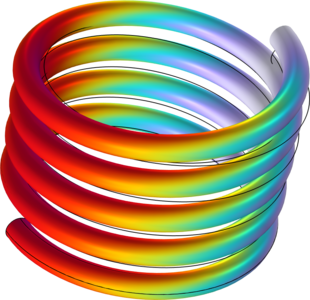# Bibliothèque d'Applications

## Loaded Spring — Using Global Equations to Satisfy Constraints

Application ID: 9999

Global equations are a way of adding an additional equation to a model. A global equation can be used to describe a load, constraint, material property, or anything else in the model that has a uniquely definable solution. In this example, a structural mechanics model of a spring is augmented by a global equation which solves for the load to achieve a desired spring displacement.This model example illustrates applications of this type that would nominally be built using the following products: